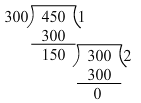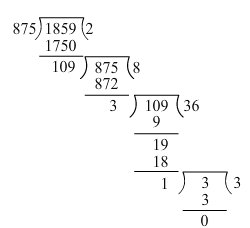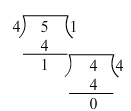×#### Thank you for registering.

One of our academic counsellors will contact you within 1 working day.

Click to Chat

1800-1023-196

+91-120-4616500

CART 0

• 0

MY CART (5)

Use Coupon: CART20 and get 20% off on all online Study Material

ITEM
DETAILS
MRP
DISCOUNT
FINAL PRICE
Total Price: Rs.

There are no items in this cart.
Continue Shopping• Complete JEE Main/Advanced Course and Test Series
• OFFERED PRICE: Rs. 15,900
• View Details

```Chapter 2: Playing With Numbers – Exercise 2.7

Question: 1

Determine the H.C.F of the following numbers by using Euclid’s algorithm (I – x):

Solution:

(i) 300 and 450

Dividend = 450 and divisor = 300Clearly, the last divisor is 150.

Hence, HCF of the given number is 150.

(ii) 399 and 437

We have dividend = 399 and divisor= 437Clearly, the last divisor is 19.

Hence, HCF of the given number is 19

(iii) 1045 and 1520

We have dividend = 1045 and divisor = 1520Clearly, the last divisor is 95.

Hence, HCF of given numbers is 95.

Question: 2

Show that the following pairs are co-prime:

Solution:

We know that two numbers are co-primes if their HCF is 1.

(i) 59 and 97

Here, dividend = 97 and divisor = 59Clearly, the last divisor is 1.

Hence, the given numbers are co-primes.

(ii) 875 and 1859

Here, dividend = 1859 and divisor = 875Clearly, the last divisor is 1.

Hence, the given numbers are co-prime.

(iii) 288 and 1375

Here, dividend = 288 and divisor = 1375Clearly, the last divisor is 1.

Hence, the given numbers are co-prime.

Question: 3

What is the H.C.F of two consecutive numbers?

Solution:

The HCF of two consecutive numbers is 1.

Example:

D = 4 and d= 5 are two consecutive numbers.

Here, we have dividend = 5 and divisor = 4Clearly, the last divisor is 1.

Hence, HCF of 4 and 5 is 1.

Question: 4

Write true (T) or false (F) for each of the following statements:

Solution:

(i) The H.CF of two distinct prime numbers is 1

True.

(ii) The H.CF of two co-prime number is 1.

True.

(iii) The H.CF of an even and an odd number is 1.

False. HCF of 6 and 9 is 3 not 1.

(iv) The H.C.F of two consecutive even numbers is 2.

True.

(v) The H.C.F of two consecutive odd numbers is 2.

False.

HCF of two consecutive odd numbers is 1.

Example: HCF of 25 and 27 is 1.
```### Course Features

• 728 Video Lectures
• Revision Notes
• Previous Year Papers
• Mind Map
• Study Planner
• NCERT Solutions
• Discussion Forum
• Test paper with Video Solution